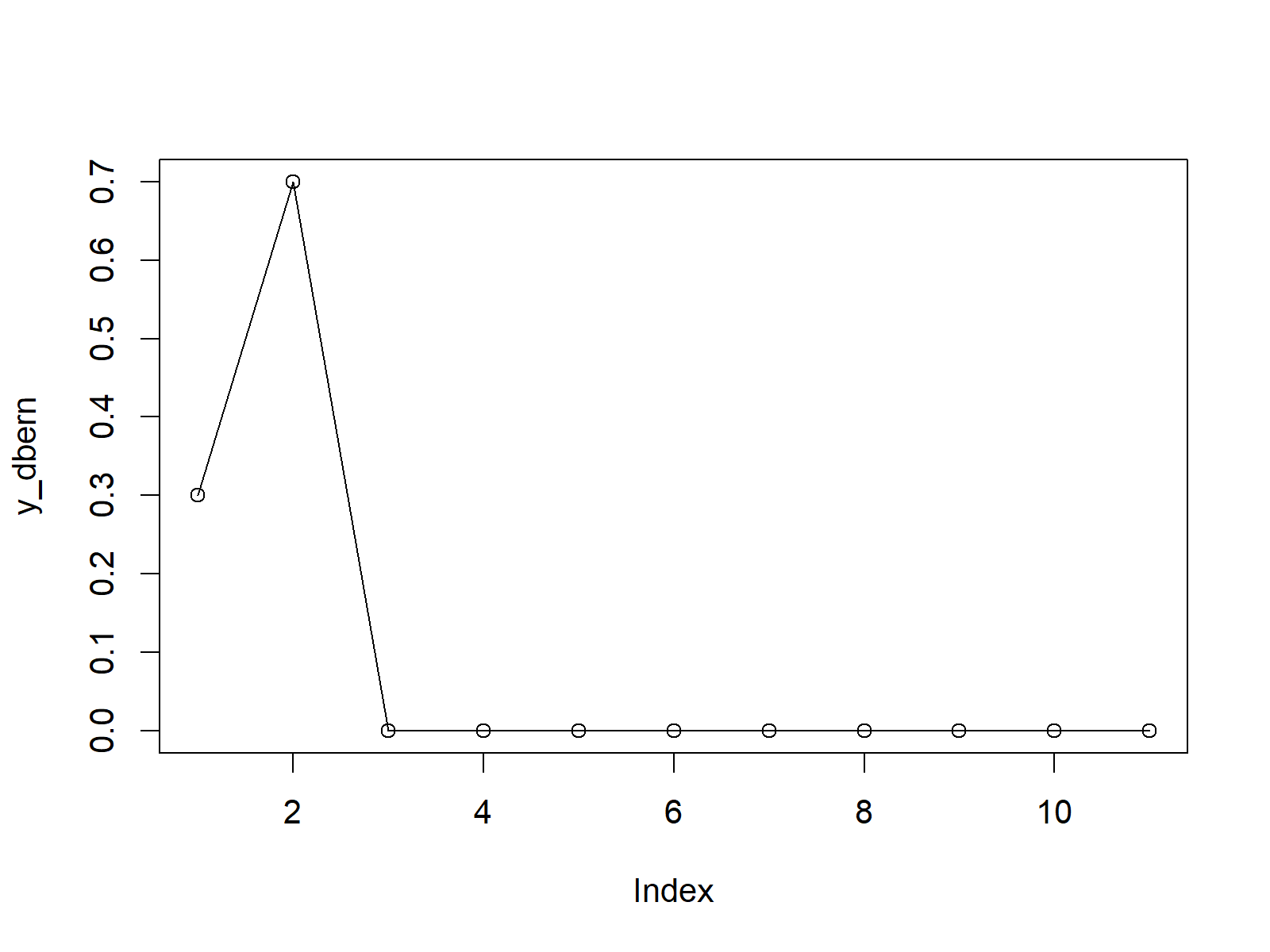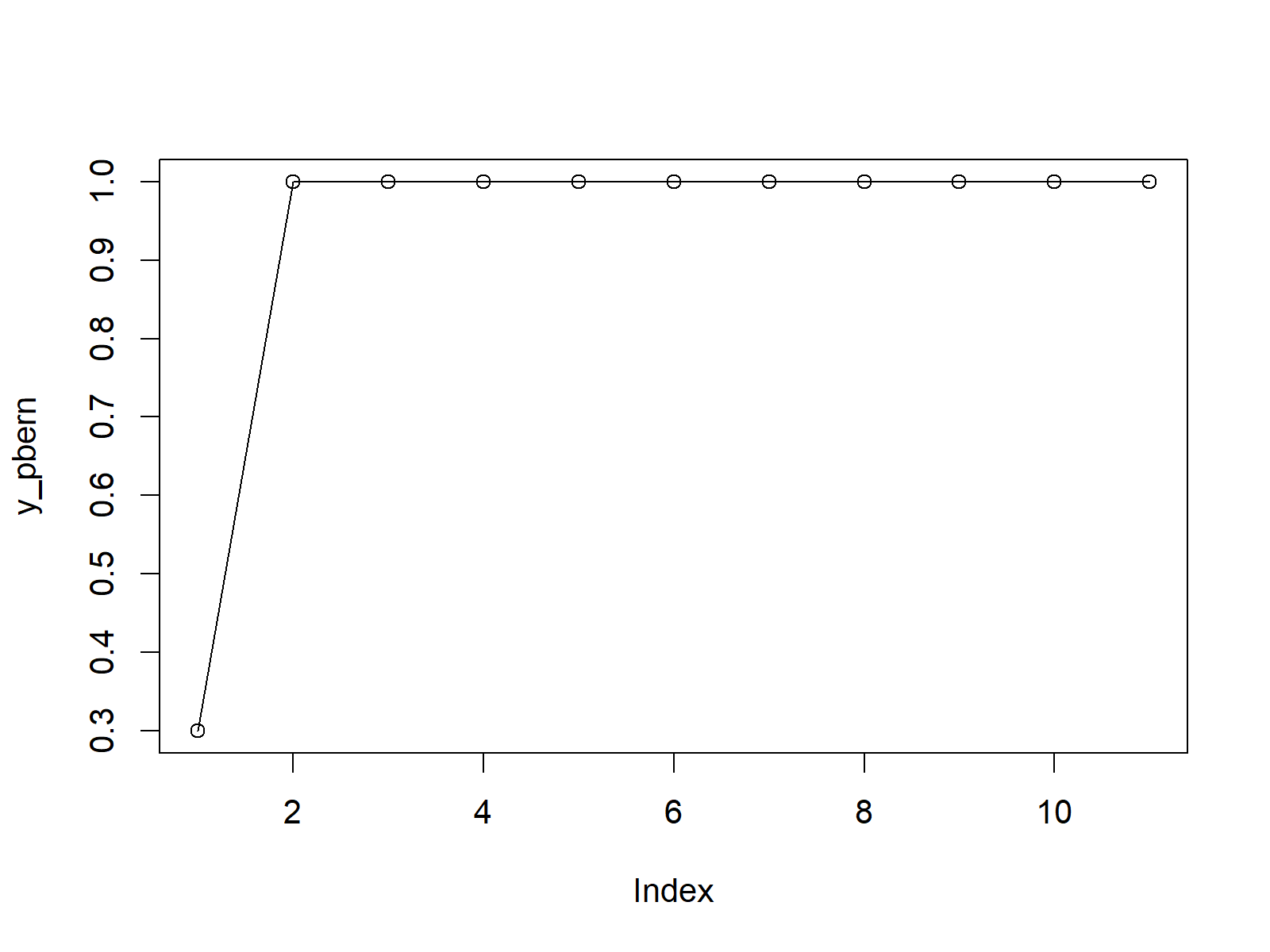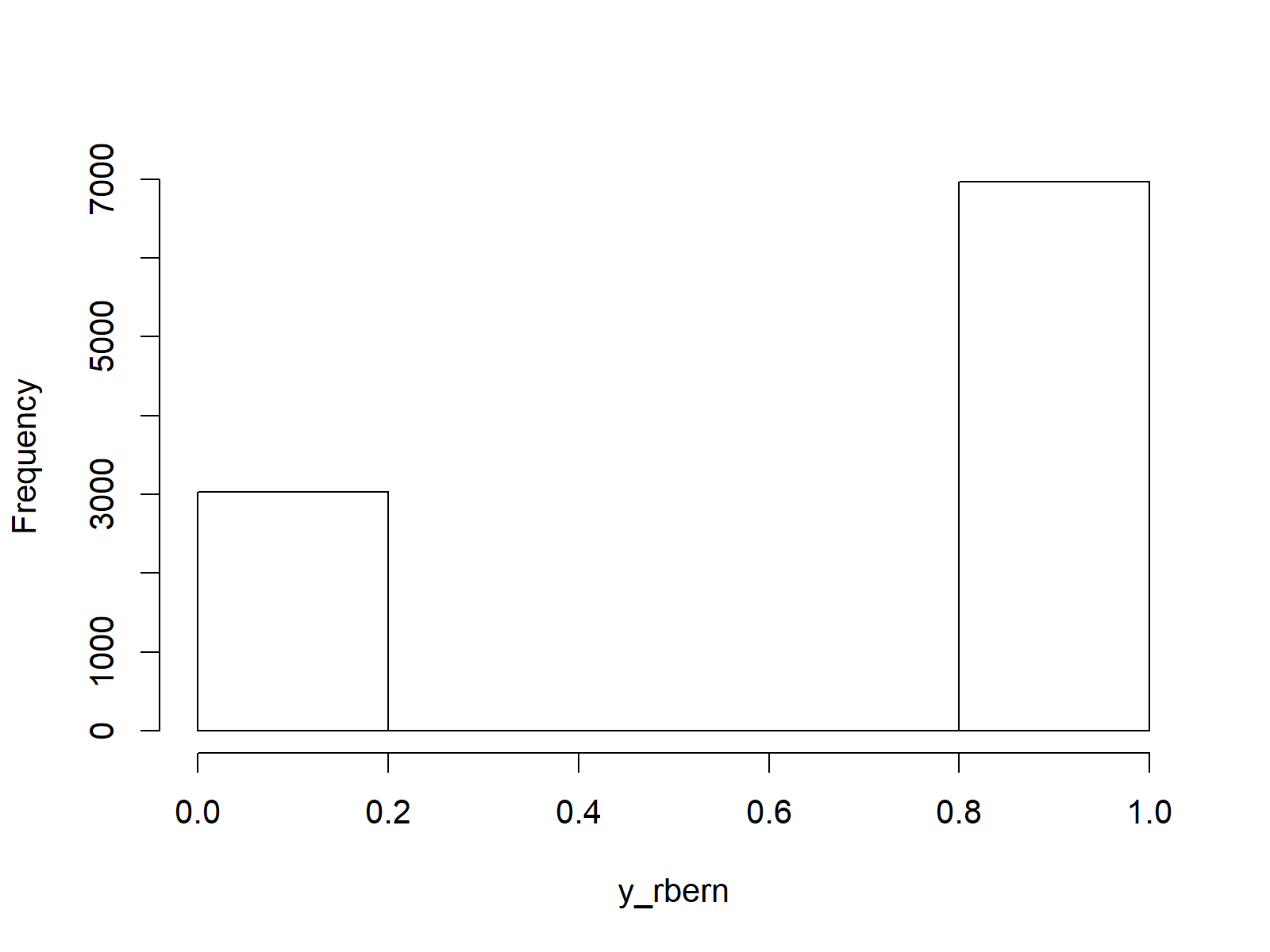# Bernoulli Distribution in R (4 Examples) | dbern, pbern, qbern & rbern Functions

In this R tutorial you’ll learn how to apply the Bernoulli distribution functions.

Let’s start right away.

## Example 1: Bernoulli Probability Density Function (dbern Function)

In the first example, I’ll show you how to draw a plot of the probability density function (PDF) of the Bernoulli distribution.

The base installation of R does not provide any Bernoulli distribution functions. For that reason, we need to install and load the Rlab add-on package first:

```install.packages("Rlab")                         # Install Rlab package

Then, we need to create a vector of quantiles in R:

`x_dbern <- seq(0, 10, by = 1)                    # Specify x-values for dbern function`

We can now apply the dbern function of the Rlab R package to our vector of quantiles in order to return the corresponding values of the Bernoulli PDF:

`y_dbern <- dbern(x_dbern, prob = 0.7)            # Apply dbern function`

If we want to draw a graphic of this distribution, we can apply the plot function as shown below:

`plot(y_dbern, type = "o")                        # Plot dbern values`Figure 1: PDF of Bernoulli Distribution in R.

## Example 2: Bernoulli Cumulative Distribution Function (pbern Function)

The R syntax for the cumulative distribution function of the Bernoulli distribution is similar as in Example 1. First, we have to create a vector of quantiles:

`x_pbern <- seq(0, 10, by = 1)                    # Specify x-values for pbern function`

Then, we can apply the pbern function to this vector:

`y_pbern <- pbern(x_pbern, prob = 0.7)            # Apply pbern function`

And finally, we can create a graph of the output of pbern with the plot function:

`plot(y_pbern, type = "o")                        # Plot pbern values`Figure 2: CDF of Bernoulli Distribution in R.

## Example 3: Bernoulli Quantile Function (qbern Function)

Example 3 shows how to create a graphic of the quantile function of the Bernoulli distribution. As a first step, we have to create a sequence of probabilities (i.e. values between 0 and 1):

`x_qbern <- seq(0, 1, by = 0.1)                   # Specify x-values for qbern function`

We can now use the qbern function to get the corresponding quantile function values for our probabilities:

`y_qbern <- qbern(x_qbern, prob = 0.7)            # Apply qbern function`

The corresponding plot can be drawn with the plot function:

`plot(y_qbern, type = "o")                        # Plot qbern values`Figure 3: Quantile Function of Bernoulli Distribution in R.

## Example 4: Generating Random Numbers (rbern Function)

To generate a set of random numbers with a Bernoulli distribution, we need to specify a seed and a sample size N first:

```set.seed(98989)                                  # Set seed for reproducibility
N <- 10000                                       # Specify sample size```

Then, we can apply the rbern function to create N Bernoulli distributed random numbers:

```y_rbern <- rbern(N, prob = 0.7)                  # Draw N random values
y_rbern                                          # Print values to RStudio console```

We can illustrate the output of the rbern function with a histogram:

```hist(y_rbern,                                    # Plot of randomly drawn density
breaks = 5,
main = "")```Figure 4: Randomly Drawn Numbers of Bernoulli Distribution in R.

## Video, Further Resources & Summary

If you need further info on the R codes of this tutorial, you may watch the following video of my YouTube channel. I illustrate the R syntax of this page in the video:

You may also have a look at the other tutorials on distributions and the simulation of random numbers in R:

In addition, I can recommend to have a look at some of the related tutorials of my homepage.

This article showed how to use the dbern, pbern, qbern, and rbern functions of the Rlab package in the R programming language. Let me know in the comments below, if you have additional questions. Furthermore, don’t forget to subscribe to my email newsletter for regular updates on the newest articles.

Subscribe to the Statistics Globe Newsletter